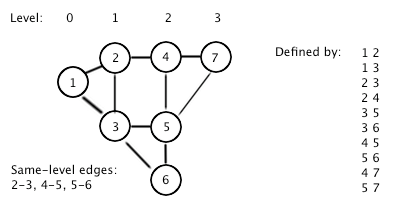DWITE Online Computer Programming Contest
November 2009
Problem 4

In a graph, each node has a "level" -- a distance to the root (start) node. One of the special properties that could be extracted, is that if there is a connection between two nodes of the same level, then there is also a cycle of odd length in the graph, which in turn gives us more properties about the structure.

The input file DATA4.txt will contain 5 sets, of input. A single positive integer 1 <= N < 50, followed by N lines describing the graph. Each line is two integers, IDs of nodes, separated by a space. Node IDs are positive integers less than 100. The root (start) node has ID 1.

The output file OUT4.txt will contain 5 lines, integer count of how many pairs of nodes have a connection, such that the shortest path from 1 to each node is equal.Sample Input (first 2 sets shown):
```3
1 2
3 2
1 3
10
1 2
1 3
2 3
2 4
3 5
3 6
4 5
5 6
4 7
5 7
```
Sample Output (first 2 sets shown):
```1
3
```